# Monoid

(Redirected from Commutative)

In algebra, a monoid is a set equipped with a binary operation satisfying certain properties similar to but less stringent than those of a group. A motivating example of a monoid is the set of positive integers with multiplication as the operation.

Formally, a monoid is a set M with a binary operation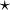$\star$ satisfying the following conditions:

• M is closed under$\star$;
• The operation$\star$ is associative;
• There is an identity element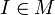$I \in M$ such that$I \star x = x = x \star I$ for all x in M.

A commutative monoid is one which satisfies the further property that$x \star y = y \star x$ for all x and y in M. Commutative monoids are often written additively: x+y rather than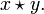$x \star y.$

An element x of a monoid is invertible if there exists an element y such that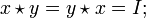$x \star y = y \star x = I;$ this is the inverse element for x and may be written as x − 1; by associativity an element can have at most one inverse. Note that x = y − 1 as well. The identity element is self-inverse and the product of invertible elements is invertible,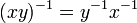$(xy)^{-1} = y^{-1} x^{-1} \,$

so the invertible elements form a group, the unit group of M.

A pseudoinverse for x is an element x + such that xx + x = x. The inverse, if it exists, is a pseudoinverse, but a pseudoinverse may exists for a non-invertible element.

A submonoid of M is a subset S of M which contains the identity element I and is closed under the binary operation.

A monoid homomorphism f from monoid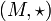$(M,{\star})$ to monoid$(N,{\circ})$ is a map from M to N satisfying

•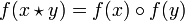$f(x \star y) = f(x) \circ f(y) \,$;
•$f(I_M) = I_N . \,$

## Examples

• The non-negative integers under addition form a commutative monoid, with zero as identity element.
• The positive integers under multiplication form a commutative monoid, with one as identity element.
• The set of all maps from a set to itself forms a monoid, with function composition as the operation and the identity map as the identity element.
• Square matrices under matrix multiplication form a monoid, with the identity matrix as the identity element; this monoid is not in general commutative.
• Every group is a monoid, by "forgetting" the inverse operation.

## Cancellation property

A monoid satisfies the cancellation property if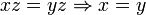$xz = yz \Rightarrow x = y \,$ and$zx = zy \Rightarrow x = y . \,$

A monoid is a submonoid of a group if and only if it satisfies the cancellation property.

## Free monoid

The free monoid on a set G of generators is the set of all words on G, the finite sequences of elements of G, with the binary operation being concatenation (juxtaposition). The identity element is the empty (zero-length) word. The free monoid on one generator g may be identified with the monoid of non-negative integers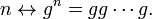$n \leftrightarrow g^n = gg \cdots g . \,$Some content on this page may previously have appeared on Citizendium.